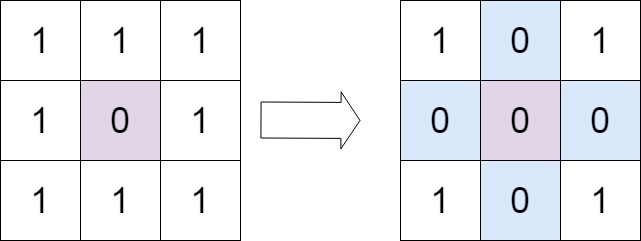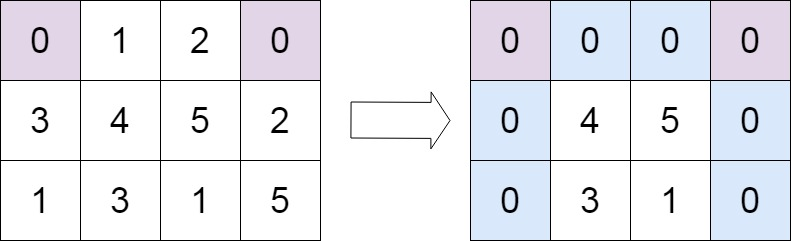# GeetCode Hub

Given an `m x n` integer matrix `matrix`, if an element is `0`, set its entire row and column to `0`'s, and return the matrix.

You must do it in place.

Example 1:```Input: matrix = [[1,1,1],[1,0,1],[1,1,1]]
Output: [[1,0,1],[0,0,0],[1,0,1]]
```

Example 2:```Input: matrix = [[0,1,2,0],[3,4,5,2],[1,3,1,5]]
Output: [[0,0,0,0],[0,4,5,0],[0,3,1,0]]
```

Constraints:

• `m == matrix.length`
• `n == matrix.length`
• `1 <= m, n <= 200`
• `-231 <= matrix[i][j] <= 231 - 1`

• A straight forward solution using `O(mn)` space is probably a bad idea.
• A simple improvement uses `O(m + n)` space, but still not the best solution.